## Example Questions

### Example Question #111 : Coordinate Geometry

What is the y-intercept of the line that goes through the points (–2, 1) and (5, 6)?

The answer cannot be determined from the given information.

67/7

17/7

0

–2/7

17/7

Explanation:

The slope can be calculated from m = (y y1)/(x– x1) = (6 – 1)/(5 + 2). Having calculated the slope, we can now use point-slope form of a line, y – y= m(x – x1), and using the second point (5, 6): y – 6 = (5/7)(x – 5). This can be rearranged into slope-intercept form to obtain: y = (5/7)x + (17/7). Because the equation is now in slope intercept form, we know that the y-intercept is 17/7.

### Example Question #1 : How To Find X Or Y Intercept

Find the x-intercept of the equation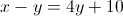–2

2

0

10

–10

10

Explanation:In order to find the x-intercept we simply let all the y's equal 0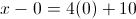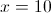### Example Question #111 : Coordinate Geometry

Quantity A:

The-intercept of the line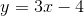Quantity B:

The-intercept of the line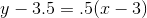Quantity B is greater

The relationship cannot be determined from the information given.

Quantity A is greater

The two quantities are equal.

The two quantities are equal.

Explanation:

The key to these quantitative comparison problems is to figure out the worth of both quantities, or figure out whether evaluating the quantities is even possible.  In this case, evaluating the quantities is a fairly straightforward case of figuring out the intercepts of two different lines, which is possible.  Therefore, you can already discount "the relationship cannot be determined from the information given".

To solve quantity A:is inform, whereis the-intercept. Therefore, the-intercept is equal toTo solve quantity B:, you have to sole for theintercept.  The quickest way to figure out the answer is to remember that theaxis exists at the line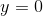, therefore to find out where the line crosses theaxis, you can setand solve for.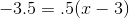-3.5 = .5x - 1.5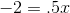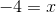Both quantity A and quantity B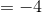, therefore the two quantities are equal.

### Example Question #1 : X And Y Intercept

What is the-intercept of the following equation?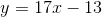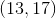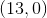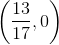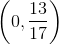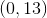Explanation:

To find the-intercept, you must plugin for.

This leaves you with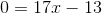.

Then you must get you by itself so you addto both sides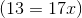.

Then divide both sides byto get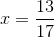.

For the coordinate point,goes first thenand the answer is.